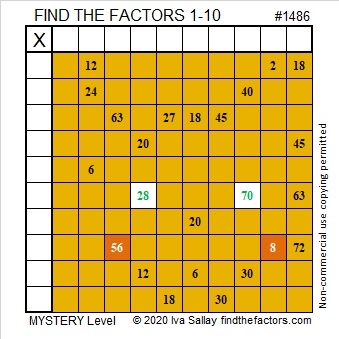# 1486 Mysterious Cat

Contents

### Today’s Puzzle:

If a cat has nine lives, how many lives do seven cats have? Where do the numbers 9 and 7 belong in this puzzle? Where do all the other numbers from 1 to 10 belong?### Factors of 1486:

• 1486 is a composite number.
• Prime factorization: 1486 = 2 × 743
• 1486 has no exponents greater than 1 in its prime factorization, so √1486 cannot be simplified.
• The exponents in the prime factorization are 1, and 1. Adding one to each exponent and multiplying we get (1 + 1)(1 + 1) = 2 × 2 = 4. Therefore 1486 has exactly 4 factors.
• The factors of 1486 are outlined with their factor pair partners in the graphic below.### More about the Number 1486:

1486 is not the sum of two squares or the difference of two squares, but it is in a Pythagorean triple:
1486-552048-552050, calculated from 2(743)(1), 743² – 1², 743² + 1²

This site uses Akismet to reduce spam. Learn how your comment data is processed.